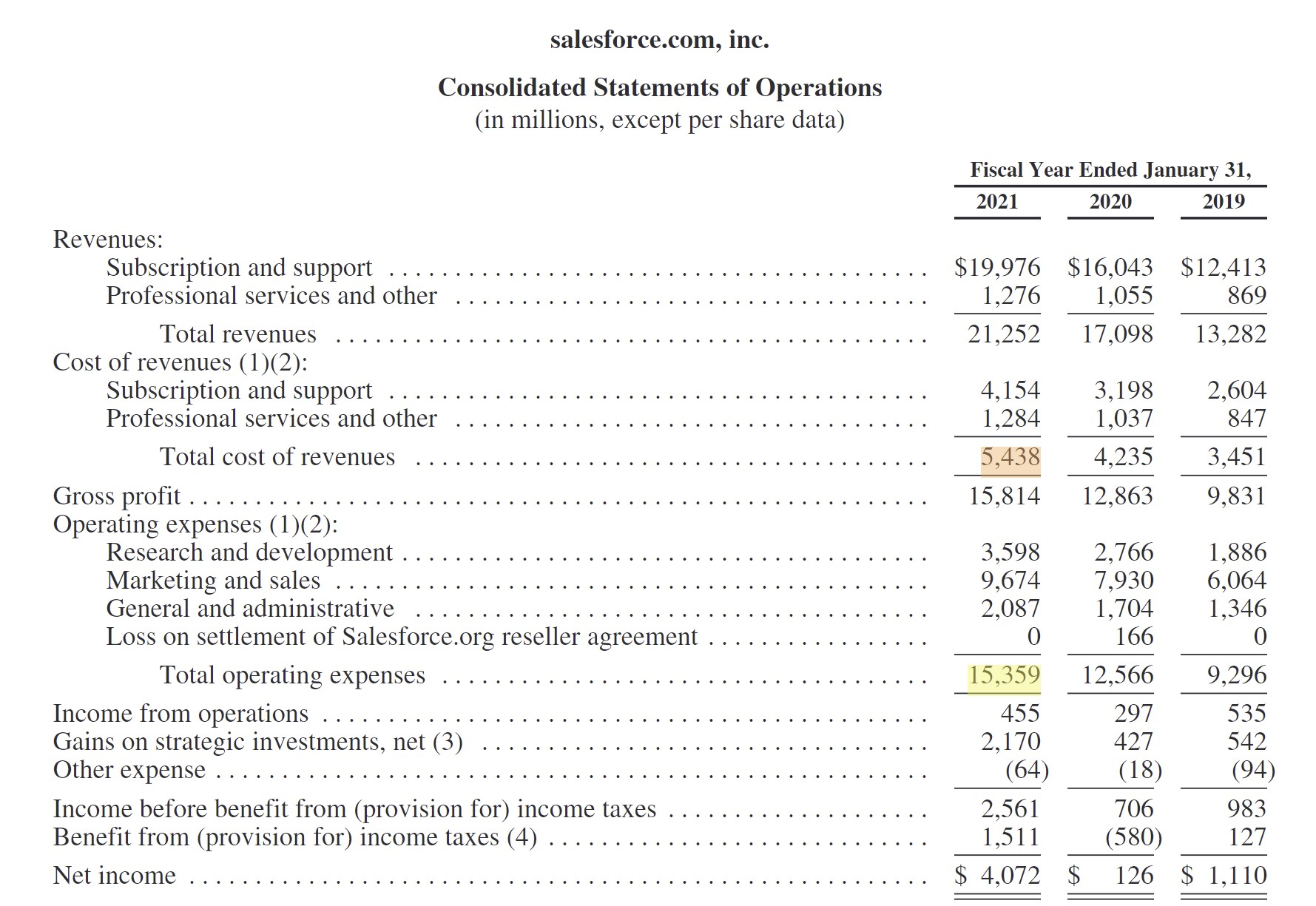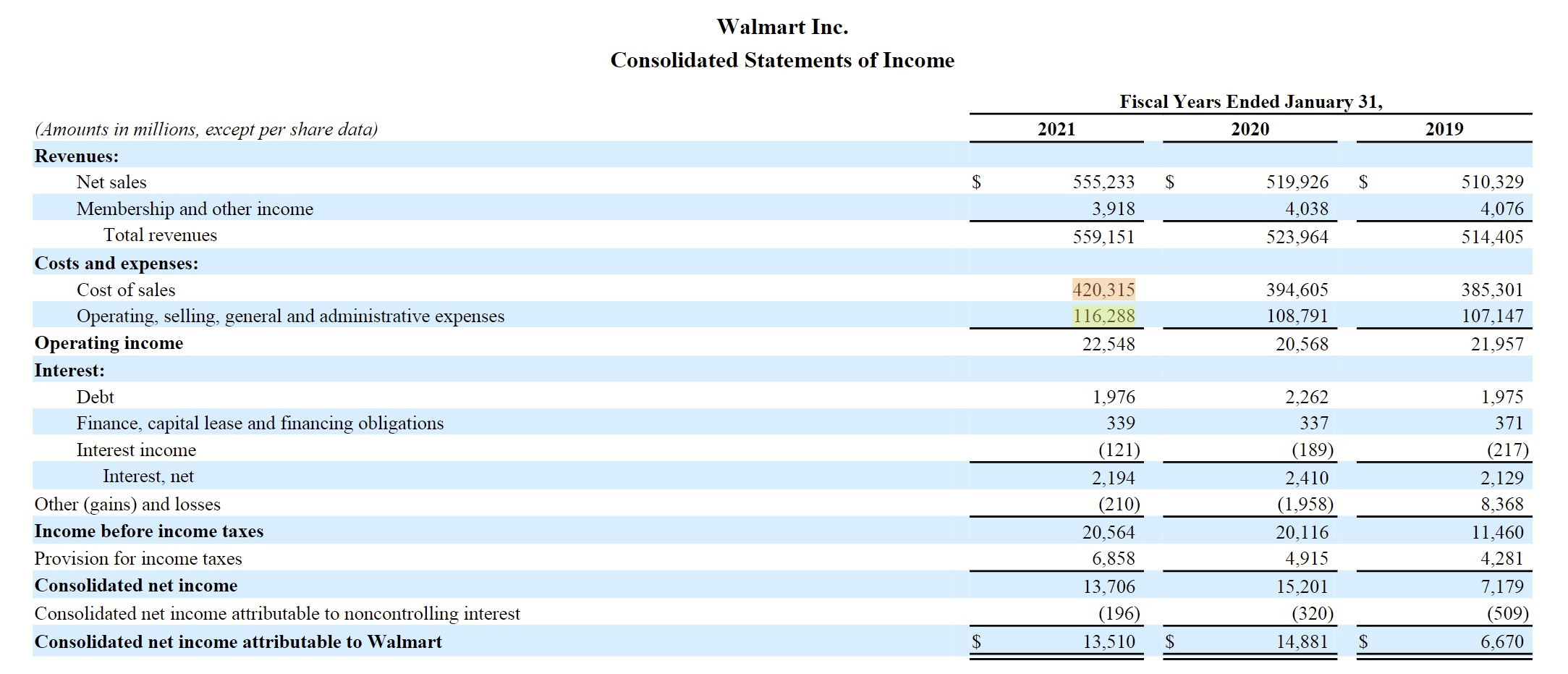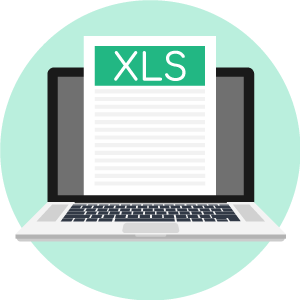# Profit Margin

Guide to Understanding Profit Margins• What is the definition of a profit margin?
• What are the common types of profit margins?
• For purposes of comparison, which profit margins are most useful?
• How does the industry influence profit margins?

## How to Calculate a Profit Margin

A profit margin is defined as a ratio that divides a profitability metric by revenue.

There are various different types of profitability metrics that could be used, such as the following:

## Profit Margin Formula

For practically all profit margins, the general “plug-in” formula is as follows.

###### Profit Margin Formula
• Profit Margin = (Profit Metric ÷ Revenue)

Typically, profit margins are denoted in percentage form, so the figure is then multiplied by 100.

## Types of Profit Margins – Summary Chart

The chart below lists the most common profit margins used for assessing companies.

Profit Margin Definition Formula
Gross Margin
• The percentage of revenue remaining once COGS have been subtracted.
• COGS are the direct costs associated with the revenue generation of a company (e.g. direct materials, direct labor).
• Gross Margin = Gross Profit / Revenue
Operating Margin
• The percentage of profitability remaining once operating expenses have been deducted from gross profit.
• Operating Margin = EBIT / Revenue
Net Profit Margin
• The percentage of accrual profitability remaining after all expenses have been subtracted.
• Net Profit Margin = Net Income / Revenue
EBITDA Margin
• The percentage of revenue remaining after all operating direct and indirect costs have been deducted – but D&A is added back due to being a non-cash expense.
• EBITDA Margin = EBITDA / Revenue

## How to Calculate Profit Margin Metrics

### Core Operating Line Items

The operating margin (or EBIT) represents the line on the income statement that splits core, ongoing business operations from non-operational line items.

Financing activities like interest on debt obligations are categorized as a non-operating expense because decisions on how to finance a company are discretionary to management (i.e. the decision to fund using debt or equity).

For comparative purposes, EBIT and EBITDA are used most frequently due to how the company’s operational performance is portrayed – while remaining independent of capital structure and taxes.

Profit margins that are independent of discretionary decisions such as the capital structure and taxes (i.e. jurisdiction-dependent) are most useful for peer comparisons.

When it comes to company-to-company comparisons, isolating the core operations of each company is important – otherwise, the values would be skewed by non-core, discretionary items.

### Non-Core Line Items

By contrast, profitability metrics that are below the operating income line (i.e. post-levered) have adjusted EBIT for non-operating income/(expenses), which are classified as discretionary and non-core to the company’s operations.

An example is the net profit margin, since non-operating income/(expenses), interest expense, and taxes are all factored into the metric.

Unlike the operating margin and EBITDA margin, the net profit margin is directly impacted by how the company is financed and the applicable tax rate.

## EBITDA vs. EBIT Margin

For purposes of comparison among different comparable companies, the two most commonly used profit margins are:

1. Operating Margin
2. EBITDA Margin

The notable difference between the two is that EBITDA is a non-GAAP measure that adds back non-cash expenses (e.g. D&A).

In particular, depreciation and amortization represent non-cash accounting conventions used to match CapEx spend with the corresponding revenue generated under the matching principle.

Besides D&A, EBITDA can also be adjusted for stock-based compensation as well as other non-recurring charges. The adjustments are done to remove the effects of non-cash expenses and non-recurring, one-time items.

## Profit Margin by Industry

Determining whether a company’s profit margin is “good” or “bad” depends on the industry in question.

Hence, comparisons among companies operating in different industries are not recommended and are likely to lead to misleading conclusions.

To provide some brief examples, software companies are well-known for exhibiting high gross margins, yet sales & marketing expenses often cut into their profitability significantly.

On the other hand, retail and wholesale stores have low gross margins due to most of their expenses being related to:

• Direct Labor
• Direct Material (i.e. Inventory)

## Salesforce – Software CRM Example

As a real-life example, we’ll look at the margin profile of Salesforce (NYSE: CRM), a cloud-based platform oriented around customer relationship management (CRM) and related applications.

In the fiscal year 2021, Salesforce had the following financials:

• Revenue: \$21.3bn
• COGS: \$5.4bn
• OpEx: \$15.4bn

Given those data points, Salesforce’s gross profit is \$15.8bn whereas its operating income (EBIT) is \$455m.

Of the core operating costs – i.e. COGS + OpEx – the corresponding % of revenue amounts were:

• COGS % Revenue: 25.6%
• OpEx % Revenue: 72.3%

Furthermore, the gross and operating margins of Salesforce in 2021 were:

• Gross Margin: 74.4%
• Operating Margin: 2.1%

As mentioned earlier, Salesforce is an example of a software company with high gross margins but with substantial operating costs, especially for sales & marketing.Salesforce Cost of Revenue and Operating Expenses (Source: 2021 10-K)

## Walmart – Retail Chain Example

Next, we’ll look at Walmart (NYSE: WMT) as a retail industry example, which we’ll contrast against our prior software industry example.

For the fiscal year 2021, Walmart had the following financial data:

• Revenue: \$559.2 bn
• COGS: \$420.3 bn
• OpEx: \$116.3bn

Therefore, Walmart’s gross profit is \$138.8bn while its operating income (EBIT) is \$22.5bn.

Just like we did for Salesforce, the operating cost breakdown (i.e. % of revenue) is as follows:

• COGS % Revenue: 75.2%
• OpEx % Revenue: 27.7%

Furthermore, the margins of Walmart were:

• Gross Margin: 24.8%
• Operating Margin: 4.0%

From our retail example, we can see how inventory and direct labor comprised the majority of Walmart’s total core expenses.Walmart Cost of Sales and Operating Expenses (Source: 2021 10-K)

## Margins by Sector – Damodaran Dataset

For those looking for a more detailed breakdown of the gross margin, operating margin, EBITDA margin, and net margin metrics for different industries, NYU Professor Damodaran has a useful resource that tracks the various average profit margins by sector:

## Profit Margin Calculator – Excel Template

We’ll now move to a modeling exercise, which you can access by filling out the form below.Submitting ...

## Profit Margin Example Calculation

Suppose we have a company with the following last twelve months (LTM) financials.

• Revenue = \$100 milion
• COGS = \$40 million
• SG&A = \$20 million
• D&A = \$10 million
• Interest = \$5 million
• Tax Rate = 20%

Using those assumptions, we can calculate the profit metrics that will be part of our margin calculations.

• Gross Profit = \$100 million – \$40 million = \$60 million
• EBITDA = \$60 million – \$20 million = \$40 million
• EBIT = \$40 million – \$10 million = \$30 million
• Pre-Tax Income = \$30 million – \$5 million = \$25 million
• Net Income = \$25 million – (\$25 million * 20%) = \$20 million

If we divide each metric by revenue, we arrive at the following profit margins for our company’s LTM performance.

• Gross Profit Margin = \$60 million / \$100 million = 60%
• EBITDA Margin = \$40 million / \$100 million = 40%
• Operating Margin = \$30 million / \$100 million = 30%
• Net Profit Margin = \$20 million / \$100 million = 20%Step-by-Step Online Course

#### Everything You Need To Master Financial Modeling

Enroll in The Premium Package: Learn Financial Statement Modeling, DCF, M&A, LBO and Comps. The same training program used at top investment banks.Inline FeedbacksLearn Financial Modeling Online

Everything you need to master financial and valuation modeling: 3-Statement Modeling, DCF, Comps, M&A and LBO.

X

The Wall Street Prep Quicklesson Series

7 Free Financial Modeling Lessons

Get instant access to video lessons taught by experienced investment bankers. Learn financial statement modeling, DCF, M&A, LBO, Comps and Excel shortcuts.